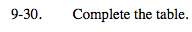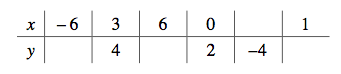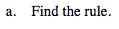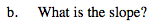### Home > MC2 > Chapter 9 > Lesson 9.1.3 > Problem9-30

9-30.Notice that there are two known coordinates: (3, 4) and (0, 2).

Now notice that for every difference of 3 in the
x-coordinate, the y-coordinate changes by 2.
Use this reasoning to fill in the rest of the table.

The difference in the y-coordinate from x = 0 to x = 3 is 2. Since 6 is twice 3, the difference in the y-coordinate from x = 0 to x = 6 is twice as much.

(−6, −2) and (−9, −4)

To find the rule, look for a pattern that can relate the
y-coordinate to the x-coordinate.

Notice that at x = 0, y = 2.
Also remember that the y-coordinate increases by 2 for every 3 units the x-coordinate increases.$\frac{\text{Change in }{\it y}}{\text{Change in }{\it x}}$

The slope describes how the values increase.
It can be written as:${\it y} = \frac{2}{3}{\it x} + 2$

You can fill in the last y-coordinate in the table by substituting 1 into the rule.

Complete the table in the eTool below to graph the points.
Click the link at right for the full version of the eTool: MC2 9-30 HW eTool